# Java数据结构和算法（六）——前缀、中缀、后缀表达式

2018/02/23 11:31

### 1、人如何解析算术表达式

如何解析算术表达式？或者换种说法，遇到某个算术表达式，我们是如何计算的：

①、求值 3+4-5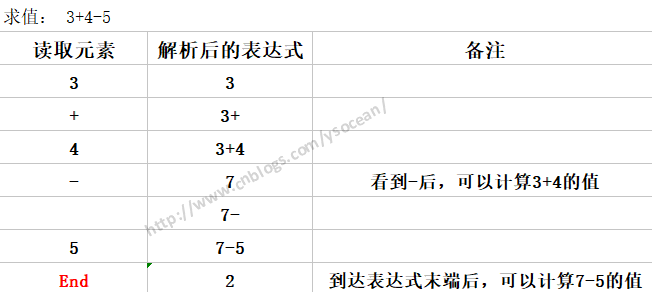这个表达式，我们在看到3+4后都不能直接计算3+4的值，知道看到4后面的 - 号，因为减号的优先级和前面的加号一样，所以可以计算3+4的值了，如果4后面是 * 或者 /，那么就要在乘除过后才能做加法操作，比如：

②、求值 3+4*5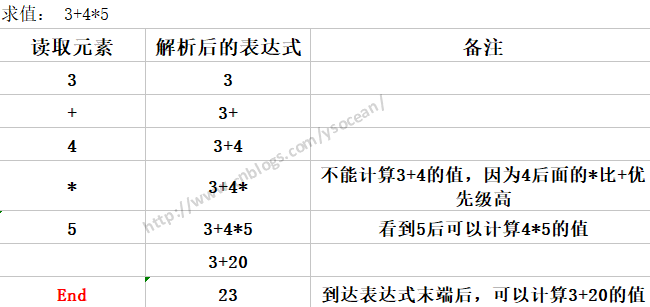这个不能先求3+4的值，因为4后面的*运算级别比前面的+高。通过这两个表达式的说明，我们可以总结解析表达式的时候遵循的几条规则：

①、从左到右读取算式。

②、已经读到了可以计算值的两个操作数和一个操作符时，可以计算，并用计算结果代替那两个操作数和一个操作符。

③、继续这个过程，从左到右，能算就算，直到表达式的结尾。

### 2、计算机如何解析算术表达式

对于前面的表达式 3+4-5，我们人是有思维能力的，能根据操作符的位置，以及操作符的优先级别能算出该表达式的结果。但是计算机怎么算？

计算机必须要向前（从左到右）来读取操作数和操作符，等到读取足够的信息来执行一个运算时，找到两个操作数和一个操作符进行运算，有时候如果后面是更高级别的操作符或者括号时，就必须推迟运算，必须要解析到后面级别高的运算，然后回头来执行前面的运算。我们发现这个过程是极其繁琐的，而计算机是一个机器，只认识高低电平，想要完成一个简单表达式的计算，我们可能要设计出很复杂的逻辑电路来控制计算过程，那更不用说很复杂的算术表达式，所以这样来解析算术表达式是不合理的，那么我们应该采取什么办法呢？

请大家先看看什么是前缀表达式，中缀表达式，后缀表达式：这三种表达式其实就是算术表达式的三种写法，以 3+4-5为例

①、前缀表达式：操作符在操作数的前面，比如 +-543

②、中缀表达式：操作符在操作数的中间，这也是人类最容易识别的算术表达式 3+4-5

③、后缀表达式：操作符在操作数的后面，比如 34+5-

上面我们讲的人是如何解析算术表达式的，也就是解析中缀表达式，这是人最容易识别的，但是计算机不容易识别，计算机容易识别的是前缀表达式和后缀表达式，将中缀表达式转换为前缀表达式或者后缀表达式之后，计算机能很快计算出表达式的值，那么中缀表达式是如何转换为前缀表达式和后缀表达式，以及计算机是如何解析前缀表达式和后缀表达式来得到结果的呢？

### 3、后缀表达式

后缀表达式，指的是不包含括号，运算符放在两个运算对象的后面，所有的计算按运算符出现的顺序，严格从左向右进行（不再考虑运算符的优先规则）。

由于后缀表达式的运算符在两个操作数的后面，那么计算机在解析后缀表达式的时候，只需要从左向右扫描，也就是只需要向前扫描，而不用回头扫描，遇到运算符就将运算符放在前面两个操作符的中间（这里先不考虑乘方类似的单目运算），一直运算到最右边的运算符，那么就得出运算结果了。既然后缀表达式这么好，那么问题来了：

#### ①、如何将中缀表达式转换为后缀表达式？

对于这个问题，转换的规则如下：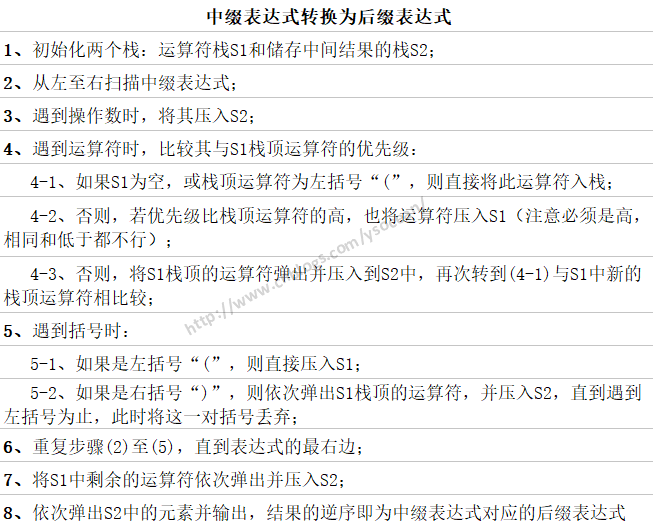一、先自定义一个栈

 1 2 3 4 5 6 7 8 9 10 11 12 13 14 15 16 17 18 19 20 21 22 23 24 25 26 27 28 29 30 31 32 33 34 35 36 37 38 39 40 41 42 43 44 45 46 47 48 49 50 51 52 53 54 55 56 `package` `com.ys.poland;`   `public` `class` `MyCharStack {` `    ``private` `char``[] array;` `    ``private` `int` `maxSize;` `    ``private` `int` `top;` `    `  `    ``public` `MyCharStack(``int` `size){` `        ``this``.maxSize = size;` `        ``array = ``new` `char``[size];` `        ``top = -``1``;` `    ``}` `    `  `    ``//压入数据` `    ``public` `void` `push(``char` `value){` `        ``if``(top < maxSize-``1``){` `            ``array[++top] = value;` `        ``}` `    ``}` `    `  `    ``//弹出栈顶数据` `    ``public` `char` `pop(){` `        ``return` `array[top--];` `    ``}` `    `  `    ``//访问栈顶数据` `    ``public` `char` `peek(){` `        ``return` `array[top];` `    ``}` `    `  `    ``//查看指定位置的元素` `    ``public` `char` `peekN(``int` `n){` `        ``return` `array[n];` `    ``}` `    `  `    ``//为了便于后面分解展示栈中的内容，我们增加了一个遍历栈的方法(实际上栈只能访问栈顶元素的)` `    ``public` `void` `displayStack(){` `        ``System.out.print(``"Stack(bottom-->top):"``);` `        ``for``(``int` `i = ``0` `; i < top+``1``; i++){` `            ``System.out.print(peekN(i));` `            ``System.out.print(``' '``);` `        ``}` `        ``System.out.println(``""``);` `    ``}` `    `  `    ``//判断栈是否为空` `    ``public` `boolean` `isEmpty(){` `        ``return` `(top == -``1``);` `    ``}` `    `  `    ``//判断栈是否满了` `    ``public` `boolean` `isFull(){` `        ``return` `(top == maxSize-``1``);` `    ``}`   `}`

二、前缀表达式转换为后缀表达式

 1 2 3 4 5 6 7 8 9 10 11 12 13 14 15 16 17 18 19 20 21 22 23 24 25 26 27 28 29 30 31 32 33 34 35 36 37 38 39 40 41 42 43 44 45 46 47 48 49 50 51 52 53 54 55 56 57 58 59 60 61 62 63 64 65 66 67 68 69 70 71 72 73 74 75 76 77 78 79 80 81 82 83 84 85 86 87 88 89 90 91 `package` `com.ys.poland;`   `public` `class` `InfixToSuffix {` `    ``private` `MyCharStack s1;``//定义运算符栈` `    ``private` `MyCharStack s2;``//定义存储结果栈` `    ``private` `String input;` `    `  `    ``//默认构造方法，参数为输入的中缀表达式` `    ``public` `InfixToSuffix(String in){` `        ``input = in;` `        ``s1 = ``new` `MyCharStack(input.length());` `        ``s2 = ``new` `MyCharStack(input.length());` `    ``}` `    ``//中缀表达式转换为后缀表达式，将结果存储在栈中返回，逆序显示即后缀表达式` `    ``public` `MyCharStack doTrans(){` `        ``for``(``int` `j = ``0` `; j < input.length() ; j++){` `            ``System.out.print(``"s1栈元素为："``);` `            ``s1.displayStack();` `            ``System.out.print(``"s2栈元素为："``);` `            ``s2.displayStack();` `            ``char` `ch = input.charAt(j);` `            ``System.out.println(``"当前解析的字符:"``+ch);` `            ``switch` `(ch) {` `            ``case` `'+'``:` `            ``case` `'-'``:` `                ``gotOper(ch,``1``);` `                ``break``;` `            ``case` `'*'``:` `            ``case` `'/'``:` `                ``gotOper(ch,``2``);` `                ``break``;` `            ``case` `'('``:` `                ``s1.push(ch);``//如果当前字符是'(',则将其入栈` `                ``break``;` `            ``case` `')'``:` `                ``gotParen(ch);` `                ``break``;` `            ``default``:` `                ``//1、如果当前解析的字符是操作数，则直接压入s2` `                ``//2、` `                ``s2.push(ch);` `                ``break``;` `            ``}``//end switch` `        ``}``//end for` `        `  `        ``while``(!s1.isEmpty()){` `            ``s2.push(s1.pop());` `        ``}` `        ``return` `s2;` `    ``}` `    `  `    ``public` `void` `gotOper(``char` `opThis,``int` `prec1){` `        ``while``(!s1.isEmpty()){` `            ``char` `opTop = s1.pop();` `            ``if``(opTop == ``'('``){``//如果栈顶是'(',直接将操作符压入s1` `                ``s1.push(opTop);` `                ``break``;` `            ``}``else``{` `                ``int` `prec2;` `                ``if``(opTop == ``'+'` `|| opTop == ``'-'``){` `                    ``prec2 = ``1``;` `                ``}``else``{` `                    ``prec2 = ``2``;` `                ``}` `                ``if``(prec2 < prec1){``//如果当前运算符比s1栈顶运算符优先级高，则将运算符压入s1` `                    ``s1.push(opTop);` `                    ``break``;` `                ``}``else``{``//如果当前运算符与栈顶运算符相同或者小于优先级别，那么将S1栈顶的运算符弹出并压入到S2中` `                    ``//并且要再次再次转到while循环中与 s1 中新的栈顶运算符相比较；` `                    ``s2.push(opTop);` `                ``}` `            ``}` `            `  `        ``}``//end while` `        ``//如果s1为空，则直接将当前解析的运算符压入s1` `        ``s1.push(opThis);` `    ``}` `    `  `    ``//当前字符是 ')' 时，如果栈顶是'(',则将这一对括号丢弃，否则依次弹出s1栈顶的字符，压入s2，直到遇到'('` `    ``public` `void` `gotParen(``char` `ch){` `        ``while``(!s1.isEmpty()){` `            ``char` `chx = s1.pop();` `            ``if``(chx == ``'('``){` `                ``break``;` `            ``}``else``{` `                ``s2.push(chx);` `            ``}` `        ``}` `    ``}`   `}`

三、测试

 1 2 3 4 5 6 7 8 9 10 `@Test` `public` `void` `testInfixToSuffix(){` `    ``String input;` `    ``System.out.println(``"Enter infix:"``);` `    ``Scanner scanner = ``new` `Scanner(System.in);` `    ``input = scanner.nextLine();` `    ``InfixToSuffix in = ``new` `InfixToSuffix(input);` `    ``MyCharStack my = in.doTrans();` `    ``my.displayStack();` `}`

四、结果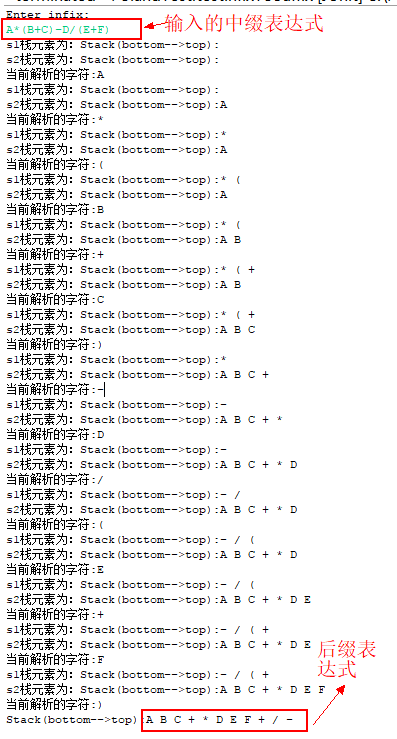五、分析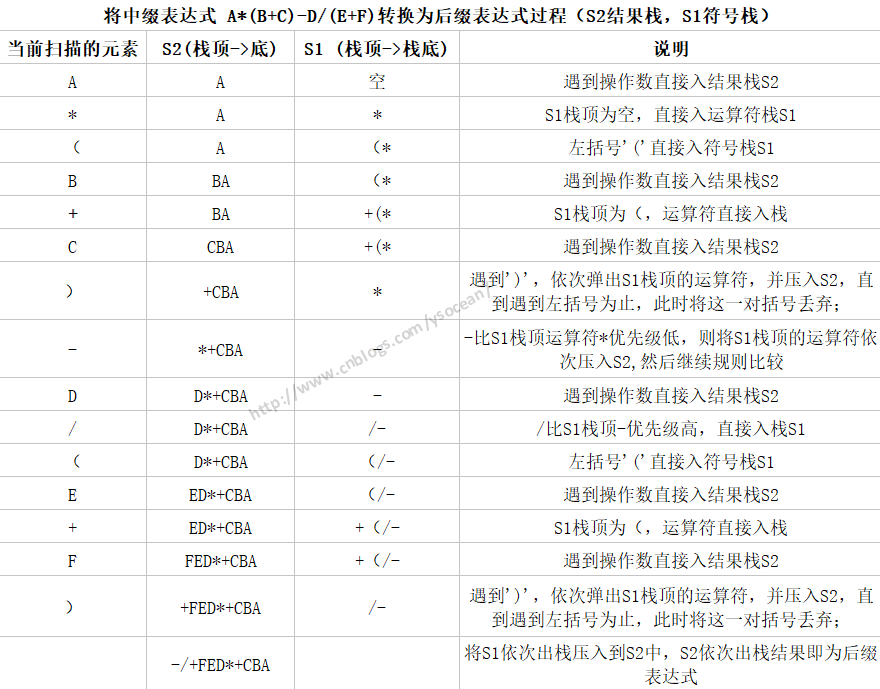#### ②、计算机如何实现后缀表达式的运算？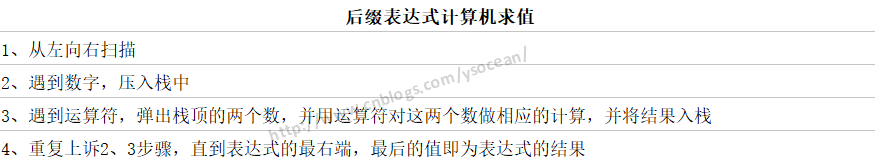1 2 3 4 5 6 7 8 9 10 11 12 13 14 15 16 17 18 19 20 21 22 23 24 25 26 27 28 29 30 31 32 33 34 35 36 37 38 39 40 41 42 43 44 45 46 47 48 49 50 51 52 53 54 `package` `com.ys.poland;`   `public` `class` `CalSuffix {` `    ``private` `MyIntStack stack;` `    ``private` `String input;` `    `  `    ``public` `CalSuffix(String input){` `        ``this``.input = input;` `        ``stack = ``new` `MyIntStack(input.length());` `        `  `    ``}` `    `  `    ``public` `int` `doCalc(){` `        ``int` `num1,num2,result;` `        ``for``(``int` `i = ``0` `; i < input.length() ; i++){` `            ``char` `c = input.charAt(i);` `            ``if``(c >= ``'0'` `&& c <= ``'9'``){` `                ``stack.push((``int``)(c-``'0'``));``//如果是数字，直接压入栈中` `            ``}``else``{` `                ``num2 = stack.pop();``//注意先出来的为第二个操作数` `                ``num1 = stack.pop();` `                ``switch` `(c) {` `                ``case` `'+'``:` `                    ``result = num1+num2;` `                    ``break``;` `                ``case` `'-'``:` `                    ``result = num1-num2;` `                    ``break``;` `                ``case` `'*'``:` `                    ``result = num1*num2;` `                    ``break``;` `                ``case` `'/'``:` `                    ``result = num1/num2;` `                    ``break``;` `                ``default``:` `                    ``result = ``0``;` `                    ``break``;` `                ``}``//end switch` `                `  `                ``stack.push(result);` `            ``}``//end else` `        ``}``//end for` `        ``result = stack.pop();` `        ``return` `result;` `    ``}` `    `  `    ``public` `static` `void` `main(String[] args) {` `        ``//中缀表达式：1*(2+3)-5/(2+3) = 4` `        ``//后缀表达式：123+*123+/-` `        ``CalSuffix cs = ``new` `CalSuffix(``"123+*523+/-"``);` `        ``System.out.println(cs.doCalc()); ``//4` `    ``}`   `}`

### 4、前缀表达式

前缀表达式，指的是不包含括号，运算符放在两个运算对象的前面，严格从右向左进行（不再考虑运算符的优先规则），所有的计算按运算符出现的顺序。

注意：后缀表达式是从左向右解析，而前缀表达式是从右向左解析。

#### ①、如何将中缀表达式转换为前缀表达式？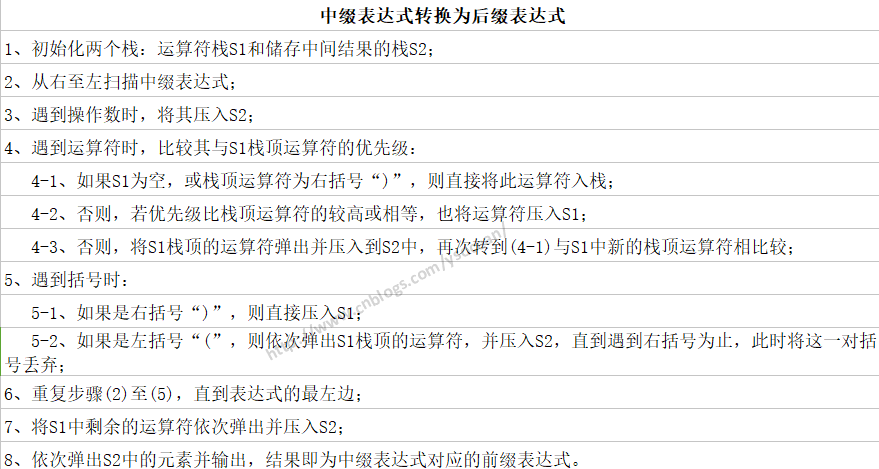#### ②、计算机如何实现前缀表达式的运算？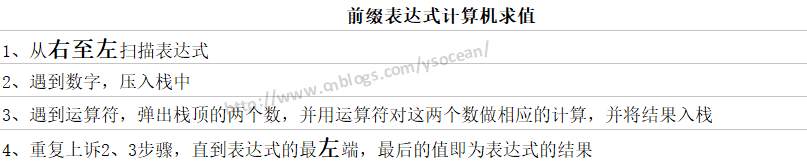1
16 收藏

### 作者的其它热门文章2018/02/23 12:29

1 评论
16 收藏
1